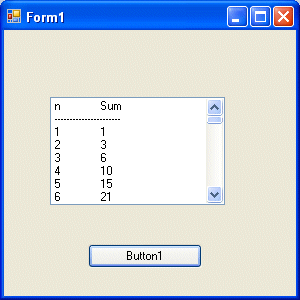# Visual Basic 2010 Lesson 11- Looping

##### [Lesson 10] << [CONTENTS] >> [Lesson 12]

In Visual Basic 2010 programming, a  sequence of instructions that is repeated until a certain condition is met is called looping.  For example, we can write VB code that adds a series of numbers until the sum exceeds a certain value or a VB program that asks the user to enter data repeatedly until he or she enters the word ‘Finish’. In Visual Basic 2010, we have three types of Loops, they are the For…..Next loop, the Do loop. and the While…..End while loop

### 11.1 Looping using the For….Next Loop

The syntax for looping using the For…Next loop is:

```For counter=startNumber to endNumber
One or more statements
Next
```

To exit a For…..Next Loop, you can place the Exit For statement within the loop; and it is typically used together with the If….Then…..statement. For its application, you can refer to example 11.1 d.

#### Example 11.1 a

```Dim counter as Integer
For counter=1 to 10
Next
```

* The program will enter number 1 to 10 into the list box.

#### Example 11.1b

```Dim counter , sum As Integer
For counter=1 to 100 step 10
sum+=counter
Next
```

*The program will calculate the sum of the numbers as follows:sum=0+10+20+30+40+……

#### Example 11.1c

```Dim counter, sum As Integer
sum = 1000
For counter = 100 To 5 Step -5
sum - = counter
Next
```

*Notice that increment can be negative.The program will compute the
subtraction as follow:
1000-100-95-90-……….

#### Example 11.1d

```Dim n as Integer
For n=1 to 10
If n>6 then
Exit For
End If
Else
Next
End If
Next
```

The process will stop when n is greater than 6.

### 11.2 Do Loop

The Do Loop structures are

a)

```Do While condition
Block of VB 2010 statements
Loop
```

b)

```Do
Block of VB2010 statements
Loop While condition
```

c)

```Do Until condition
Block of VB2010 statements
Loop
```

d)

```Do
Block of VB 2010 statements
Loop Until condition
```

Sometimes we need to exit a loop prematurely because a condition is fulfilled. The keyword to use is known as Exit Do.

Let’s examine the following examples

#### Example 11.2(a)

```Do while counter <=1000
TextBox1.Text=counter
counter +=1Loop
```

* The above example will keep on adding until counter >1000.

The above example can be rewritten as

```Do
TextBox1.Text=counter
counter+=1
Loop until counter>1000
```

#### Example 11.2(b)

```Private Sub Button1_Click(ByVal sender As System.Object, ByVal e As System.EventArgs) Handles Button1.Click

Dim sum, n As IntegerListBox1.Items.Add("n" & vbTab & "Sum")
Do
n += 1
sum += n
If n = 100 Then
Exit Do
End If
Loop
End Sub
```

In the above example, we find the arithmetic summation of 1+2+3+4+……+100. In the design stage, you need to insert a ListBox into the form for displaying the output.The program uses the Add method to populate the ListBox. The statement

`ListBox1.Items.Add("n" & vbTab & "sum")`

will display the headings in the ListBox, where it uses the vbTab function to create a space between the heading n and sum.

The statement

`ListBox1.Items.Add(n & vbTab & sum)`

will list the number n and the values of the arithmetic summation.

The output is displayed below:### 11.3 The While…End While Loop

The structure of a While….End While is very similar to the Do Loop. The syntax is as follows:

```While condition
Statements
End While
```

The above loop means that while the condition is not met, the loop will go on.The loop will end when the condition is met.

#### Example 11.3

```Dim sum, n As Integer
Private Sub Button1_Click(ByVal sender As System.Object, ByVal e As System.EventArgs) Handles Button1.Click

Dim sum, n As Integer
While n <> 100
n += 1
sum = sum + n